Geometry

# Volume: Level 2 Challenges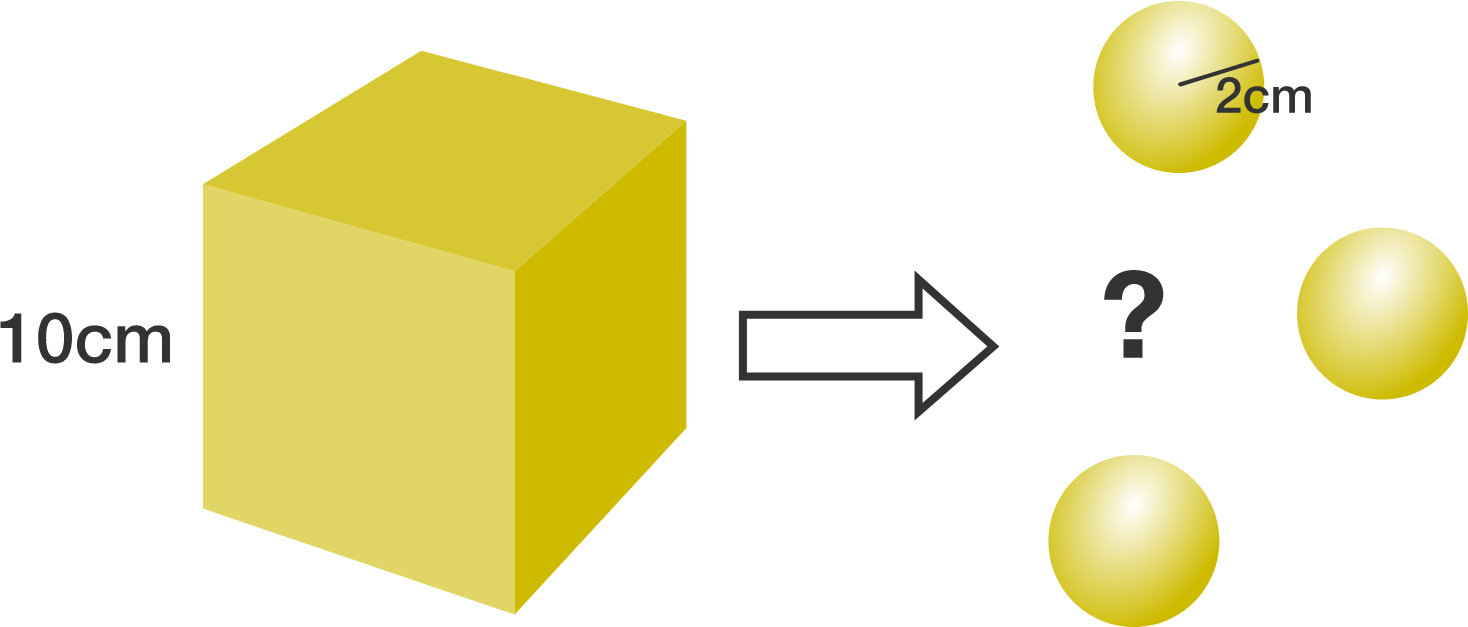A solid golden cube with side 10 cm is melted into solid golden spheres of radius 2 cm. What is the maximum number of golden spheres that can be made?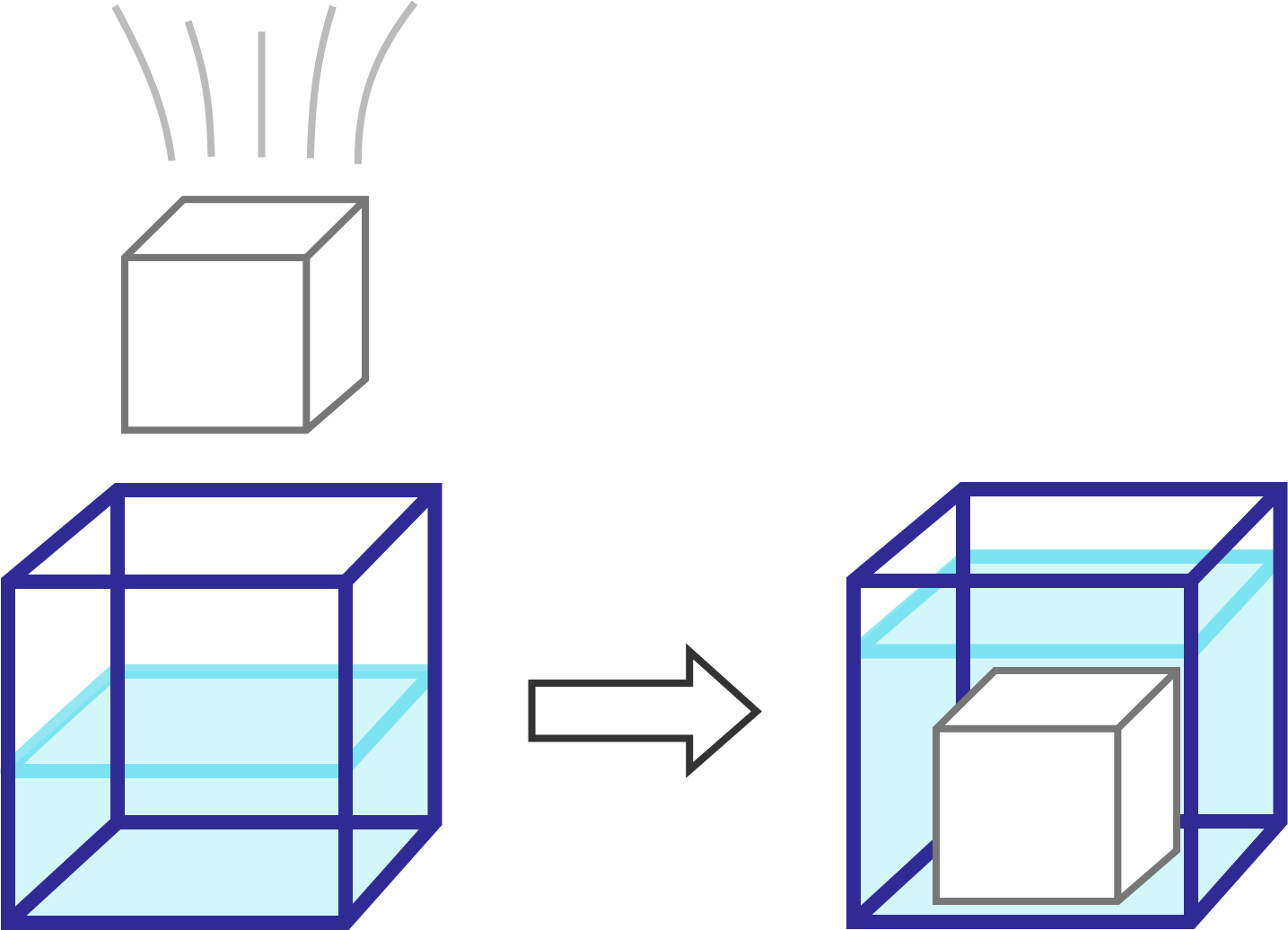A cube with a side length of 1008 units is dropped and completely submerged in a cube-shaped container.

If the water level in the cube-shaped container rises 252 units. Find the length of a side of the cube-shaped container.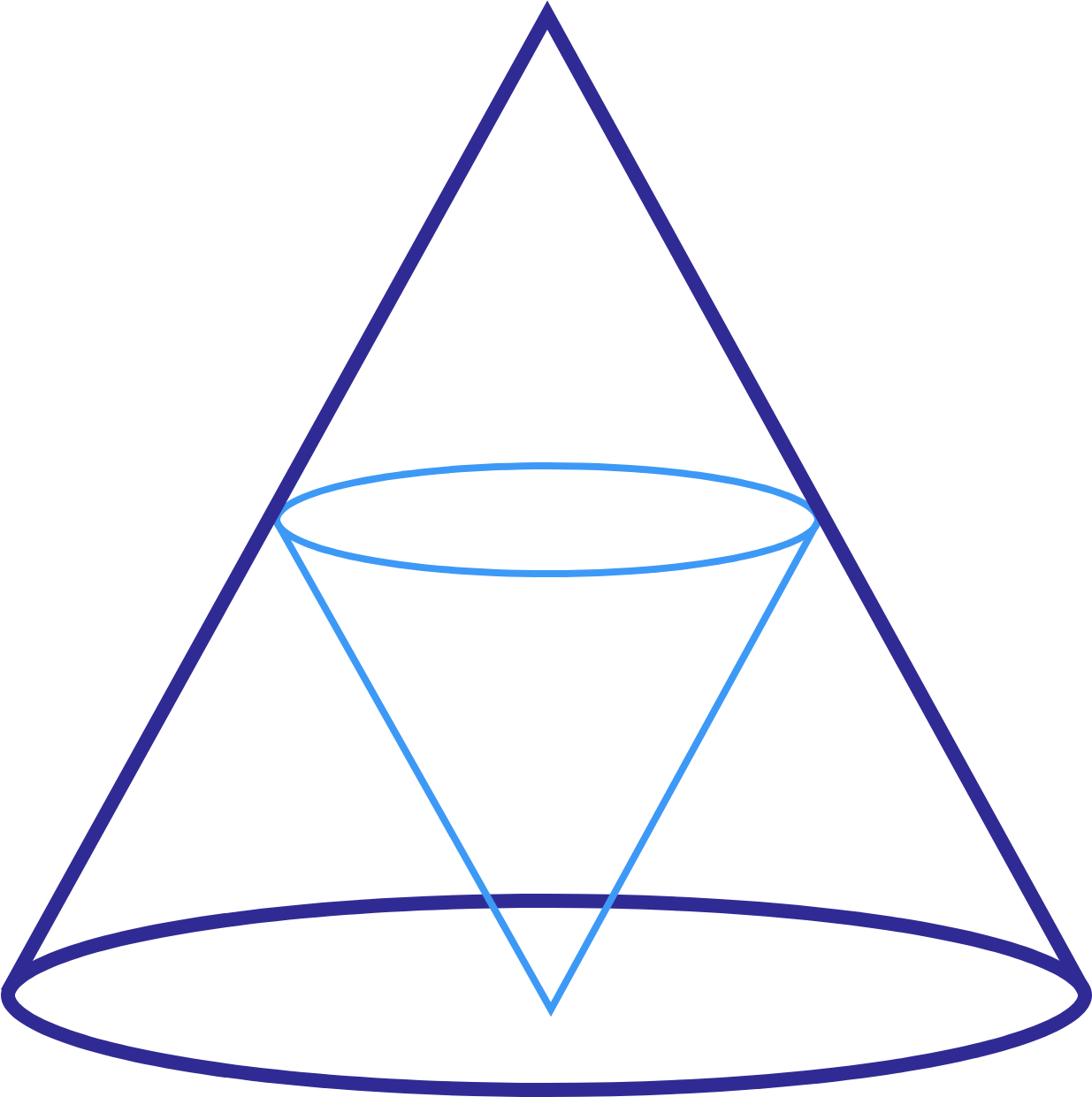A cone has a volume of 48 units.

Inside the cone we place a second, smaller cone, as shown in the drawing.

• The tip of the small cone touches the center of the large cone's base.

• The circumference of the small cone's base lies on the large cone's mantle, precisely halfway up the large cone.

How much is the volume of the smaller cone?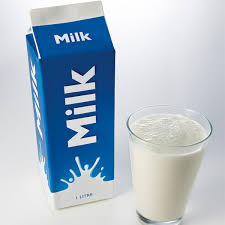You have bought 1200 cm$^3$ of milk. You poured all of the milk into a container whose base is a rectangle measuring 12cm $\times$ 10cm.

Then you decided that you would heat up some milk and you poured some of it into a pot that has a bottom area of 80 centimeter squared. Then you discovered that the height of milk in both containers are the same. How much milk, in cm$^3$, is in the pot?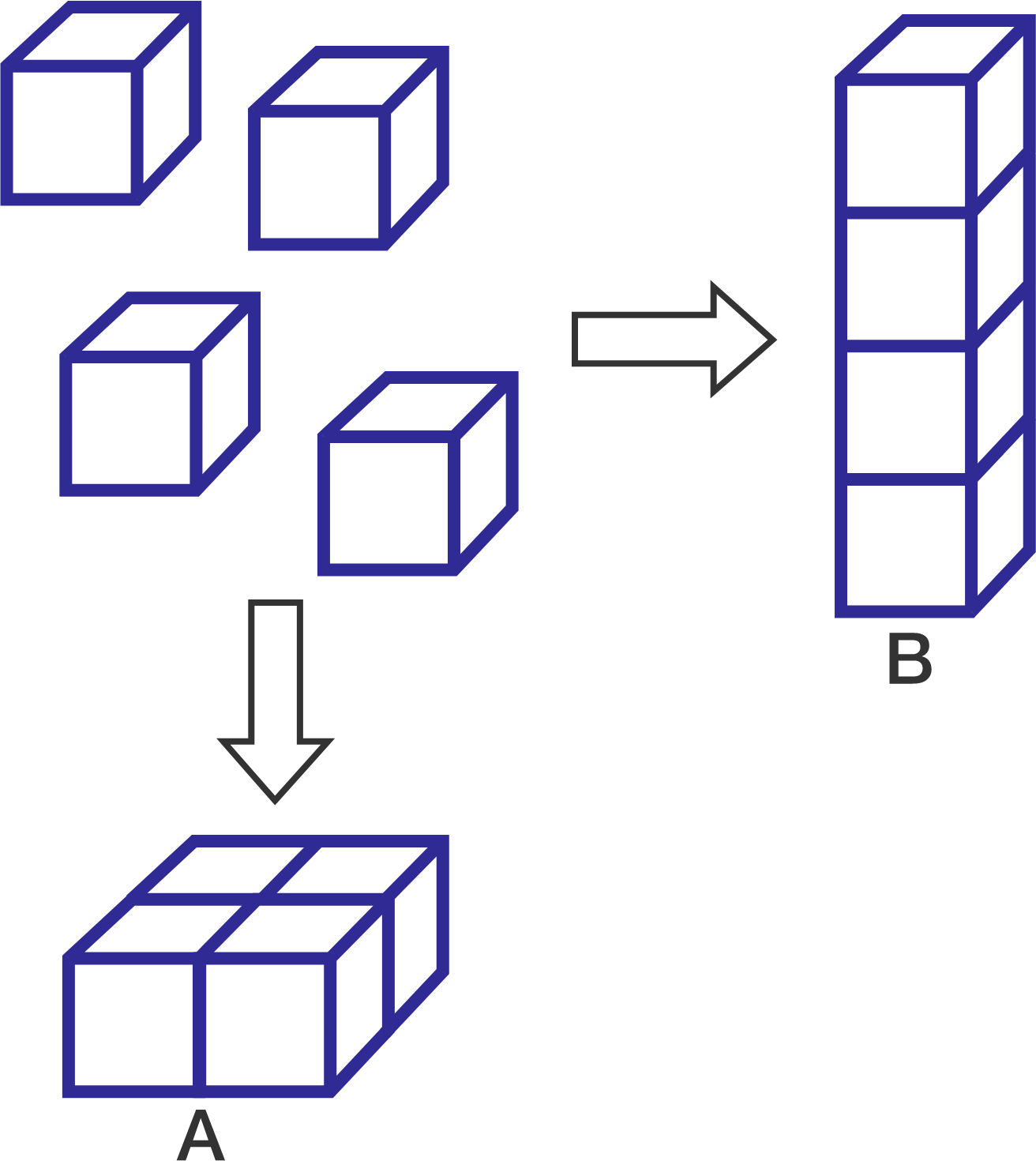4 cubes are arranged to form cuboid $A$ and cuboid $B$. Cuboid $B$'s surface area is 288 larger than cuboid $A$'s surface area.

Find the volume of cuboid $A$.

×